Question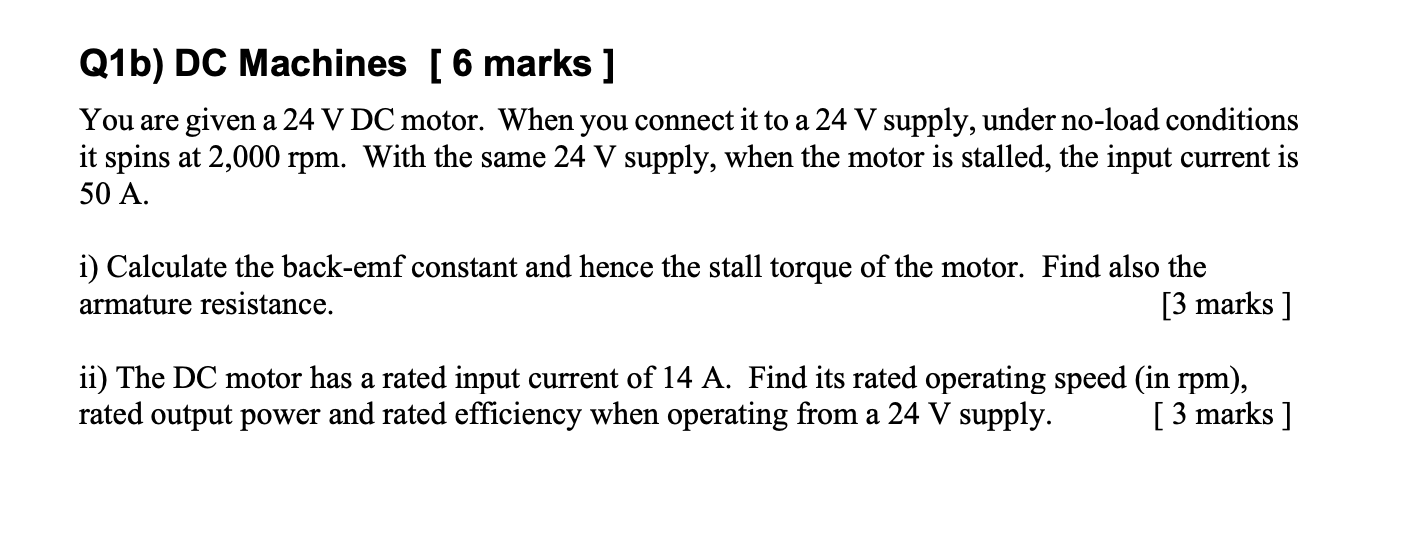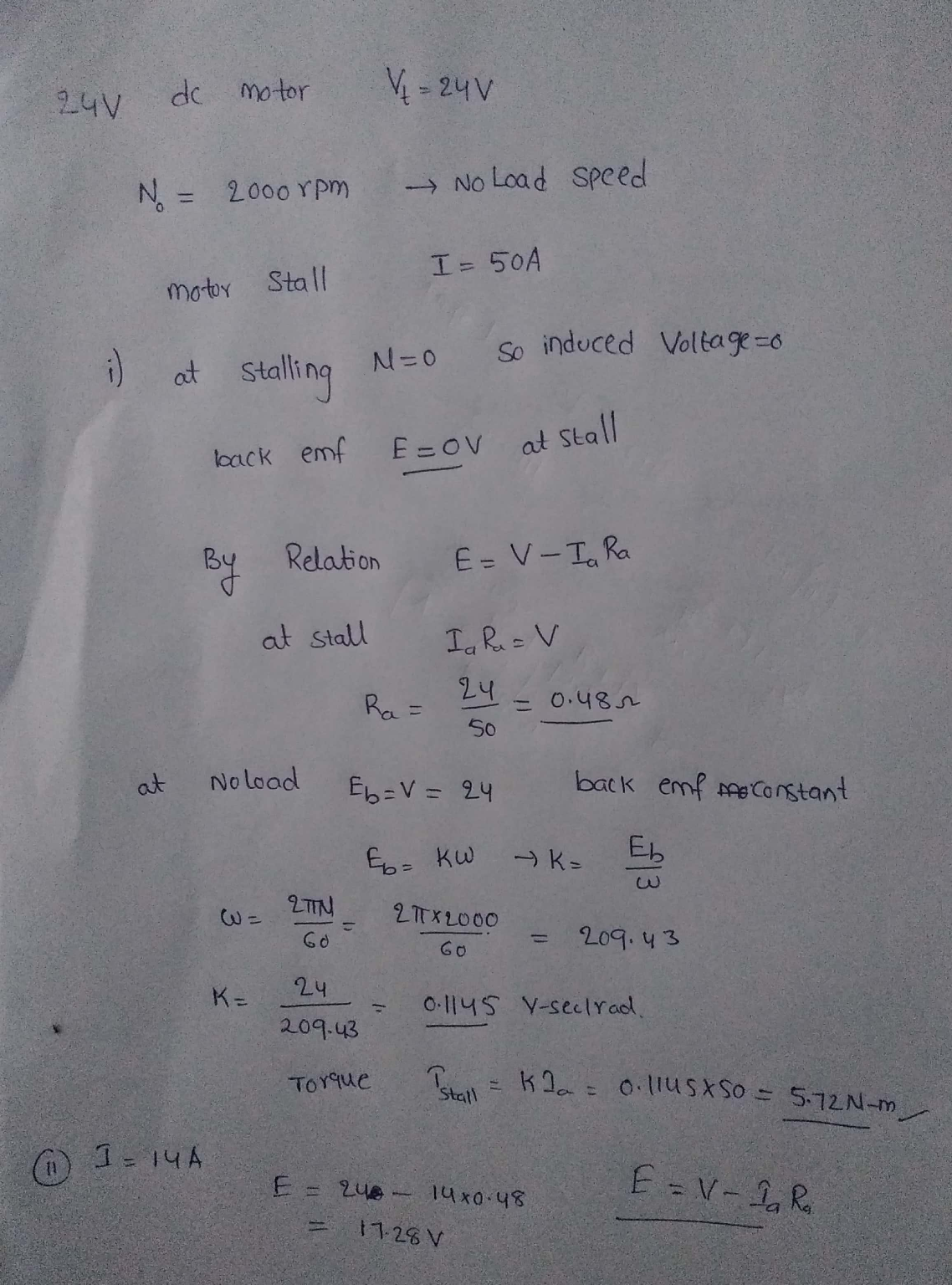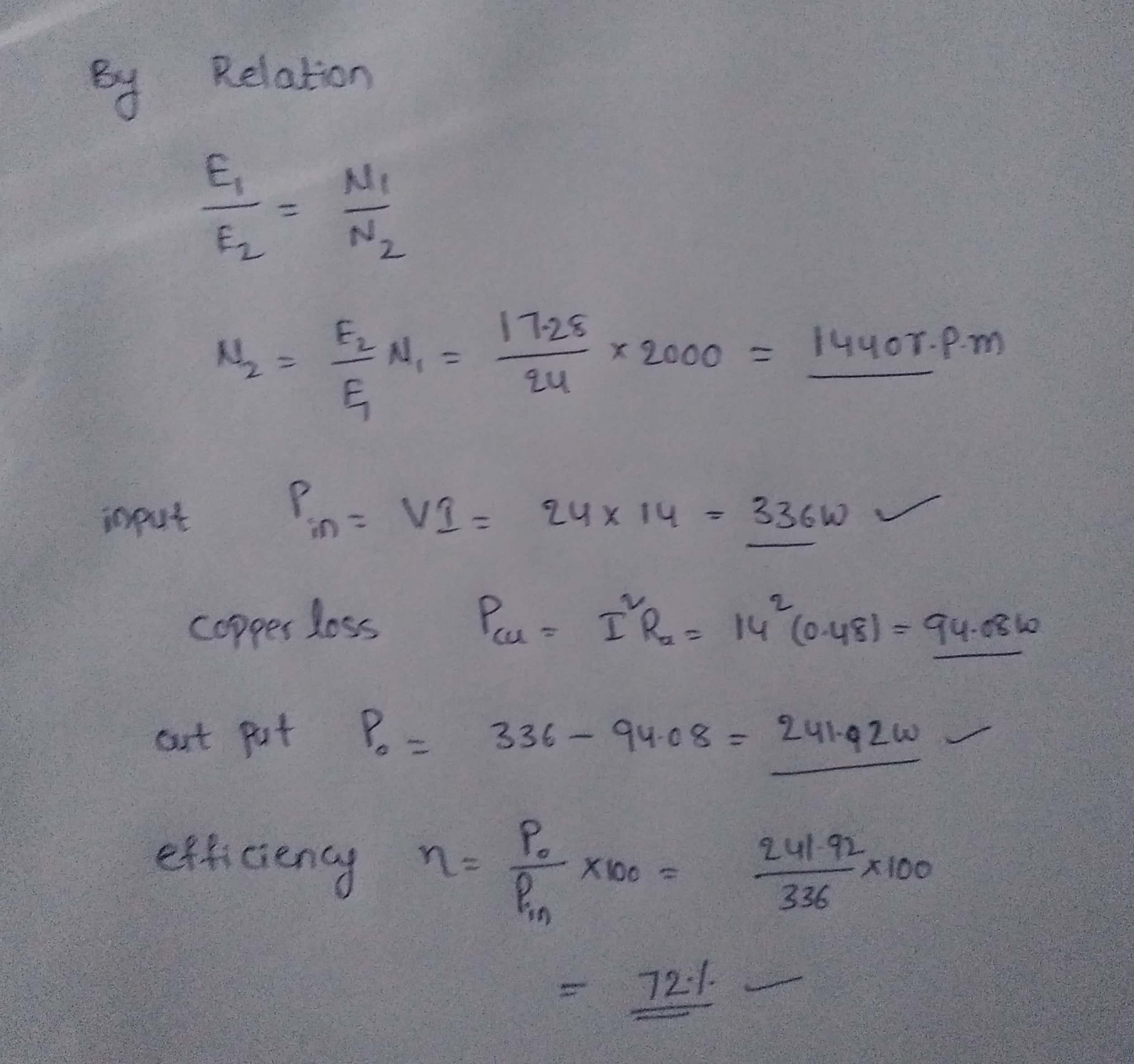#### Earn Coins

Coins can be redeemed for fabulous gifts.

Similar Homework Help Questions
• ### Q5.8：The specifications of machine 5.8. For the machine in the previous question, calculate (a) its no-load speed (b)its rated efficiency-olcoxL-n/nN (c) the following when the speed is ze...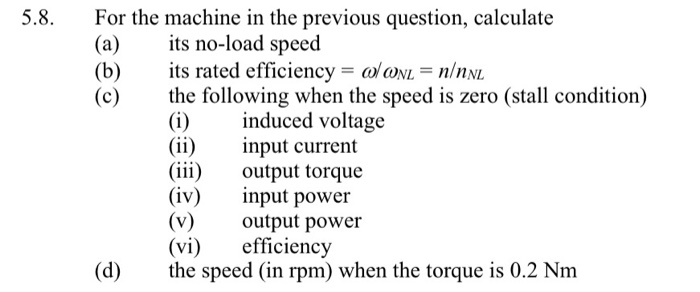Q5.8：The specifications of machine 5.8. For the machine in the previous question, calculate (a) its no-load speed (b)its rated efficiency-olcoxL-n/nN (c) the following when the speed is zero (stall condition) (i)induced voltage (ii) nput current (ii) output torque (iv) ut power (v)output power (vi) efficiency (d) he speed (in rpm) when the torque is 0.2 Nm 5 A DC machine has a no-load speed of 10,000 rpm and a rated speed of 8,000 rpm when operating with its rated supply...

• ### 5.7. A DC machine has a back-emf constant of 0.24 V/(rad/s) and a resistance of 0.5...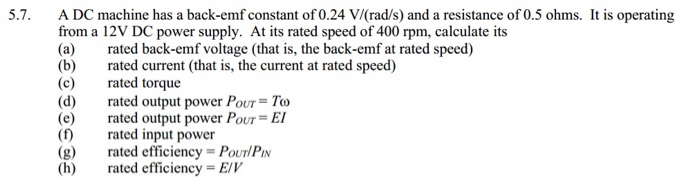5.7. A DC machine has a back-emf constant of 0.24 V/(rad/s) and a resistance of 0.5 ohms. It is operating from a 12V DC power supply. At its rated speed of 400 rpm, calculate its (a) rated back-emf voltage (that is, the back-emf at rated speed) (b)rated current (that is, the current at rated speed) (c) rated torque (d) rated output power POUT= To (e) rated output power POUT = EI (f) rated input power (g) rated efficiency-PouTlPi (h) rated...

• ### Problem 3: DC machine A shunt DC motor rated at V,'. 300 V, with field resistance...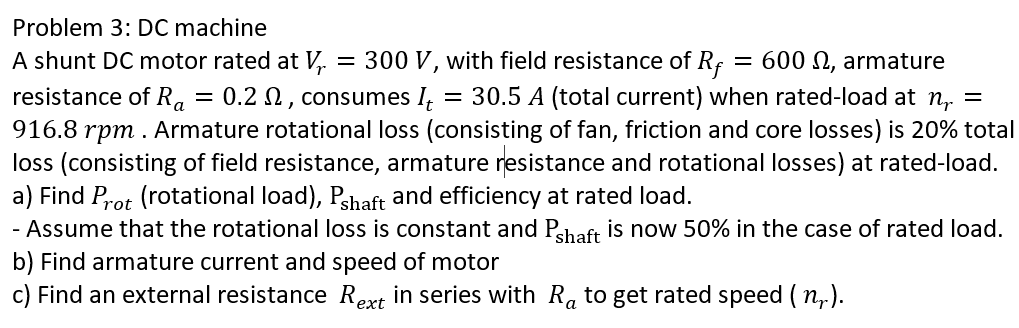Problem 3: DC machine A shunt DC motor rated at V,'. 300 V, with field resistance of Rf 600 0, armature 0.2 , resistance of R, 30.5 A (total current) when rated-load at n, consumes 916.8 rpm. Armature rotational loss (consisting of fan, friction and core loss (consisting of field resistance, armature a) Find Prot (rotational load), Pshaft and efficiency at rated load. - Assume that the rotational loss is constant and P,aft is now 50% in the case of...

• ### 6. The speed of a 150 HP, 650 V, 1750 rpm, separately excited DC motor is...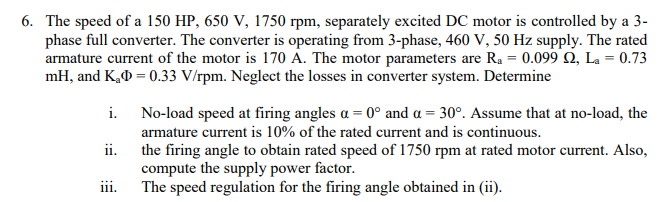6. The speed of a 150 HP, 650 V, 1750 rpm, separately excited DC motor is controlled by a 3- phase full converter. The converter is operating from 3-phase, 460 V, 50 Hz supply. The rated armature current of the motor is 170 A. The motor parameters are Ra = 0.099 2, La = 0.73 mH, and K.D=0.33 V/rpm. Neglect the losses in converter system. Determine i. No-load speed at firing angles a = 0º and a = 30°. Assume...

• ### Question 3: DC motors can be simplistically modeled as shown in Figure 5 MoTor back emf Vi: +1 Re...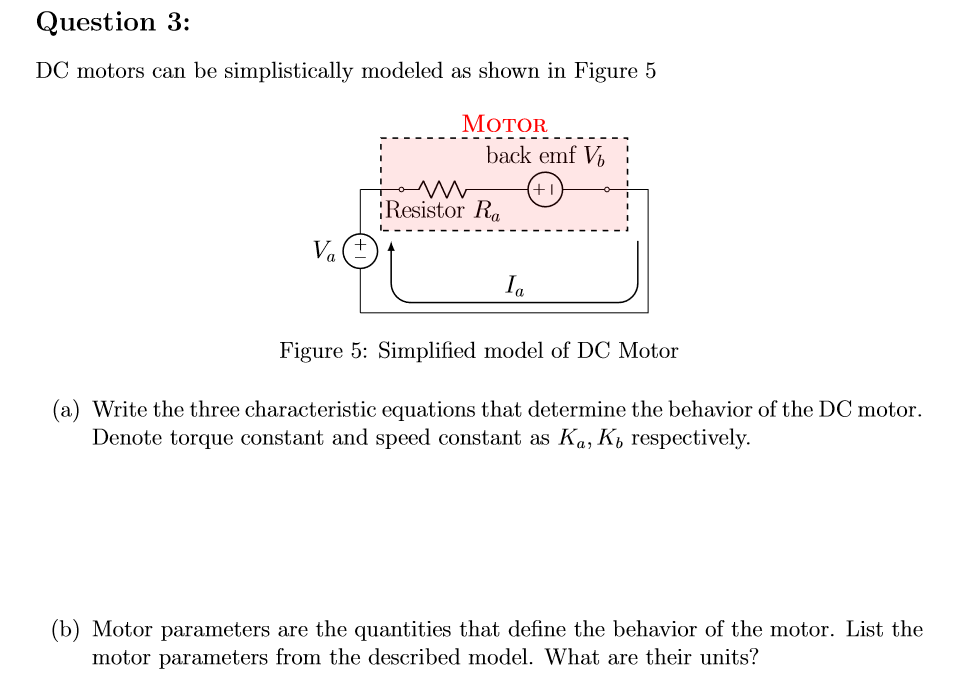Question 3: DC motors can be simplistically modeled as shown in Figure 5 MoTor back emf Vi: +1 Resistor Ra Ia Figure 5: Simplified model of DC Motor (a) Write the three characteristic equations that determine the behavior of the DC motor. Denote torque constant and speed constant as Ka, K, respectively. (b) Motor parameters are the quantities that define the behavior of the motor. List the motor parameters from the described model. What are their units? (c) For given...

• ### A DC motor, when supplied from a 211 V power supply is observed to rotate under...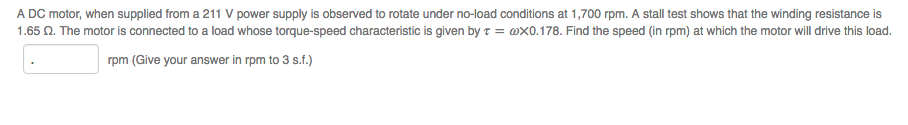A DC motor, when supplied from a 211 V power supply is observed to rotate under no-load conditions at 1,700 rpm. A stall test shows that the winding resistance is 1.65 ? The motor is connected to a load whose torque-speed characteristic is given by ? = ?×0.178. Find the speed (in rpm) at which the motor will drive this load. rpm (Give your answer in rpm to 3 s.f.)

• ### need the answer for the question no. 1 ENGR 113 Homework DC Motor Objectives Plot the...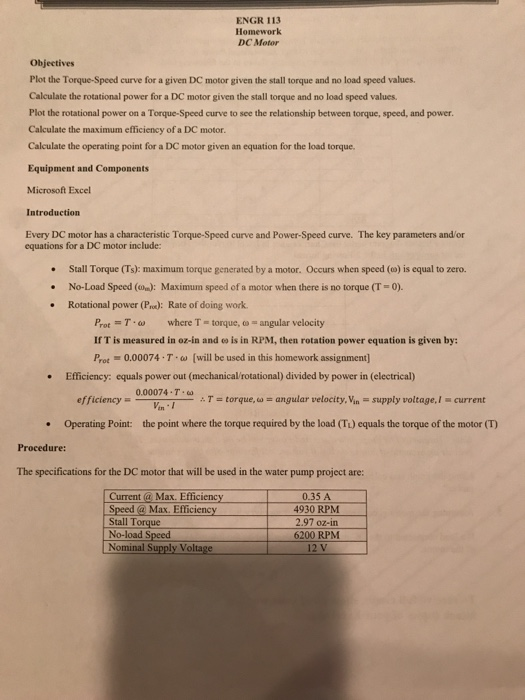need the answer for the question no. 1 ENGR 113 Homework DC Motor Objectives Plot the Torque-Speed curve for a given DC motor given the stall torque and no load speed values Calculate the rotational power for a DC motor given the stall torque and no load speed values Plot the rotational power on a Torque-Speed curve to see the relationship between torque, speed, and power Calculate the maximum efficiency of a DC motor. Calculate the operating point for a...

• ### 1. A 300 V compound motor has armature resistance 0.18 Ω, series field resistance 0.3 Ω...

1. A 300 V compound motor has armature resistance 0.18 Ω, series field resistance 0.3 Ω and shunt field resistance 100 Ω. The rotational losses are 200 W. On full load the line current is 25 A and the motor runs at 1800 rpm. Determine: (i) the developed mechanical power (ii) the output power (iii) the output torque (iv) the efficiency at full load 2. A 120 V series motor has 0.2 Ω field resistance. On full load, the line...

• ### For a PMDC motor powered by a 12 V DC source, the no-load speed and stall...

For a PMDC motor powered by a 12 V DC source, the no-load speed and stall torque are 3800 RPM and 30 mNm, respectively. If the motor is running at 2500 RPM, find a) Shaft torque (Tshaft), b) Rotor current (Im), c) Back emf (Eb), d) Electrical power consumed, e) Shaft power, and f) Power loss in rotor coil.

• ### QUESTION 1 A) (10 marks) A 10 kW, 250 V DC self-excited shunt generator, with an...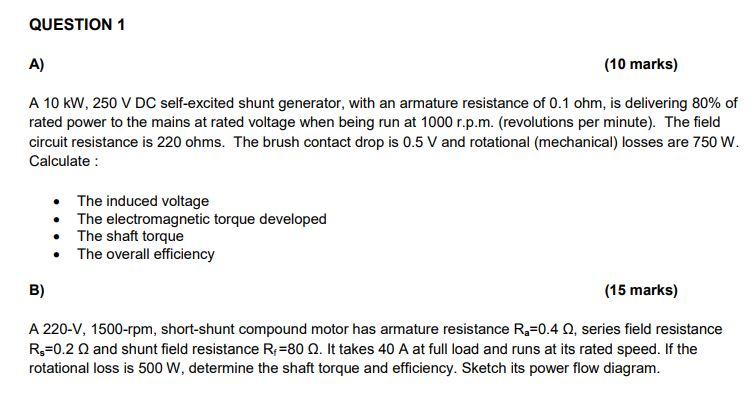QUESTION 1 A) (10 marks) A 10 kW, 250 V DC self-excited shunt generator, with an armature resistance of 0.1 ohm, is delivering 80 % of rated power to the mains at rated voltage when being run at 1000 r.p.m. (revolutions per minute). The field circuit resistance is 220 ohms. The brush contact drop is 0.5 V and rotational (mechanical) losses are 750 W Calculate The induced voltage The electromagnetic torque developed The shaft torque The overall efficiency B) (15...